#臭大佬

## 布隆过滤器

#### 使用场景

• 解决Redis缓存穿透问题（面试重点）
• 邮件过滤，使用布隆过滤器来做邮件黑名单过滤
• 对爬虫网址进行过滤，爬过的不再爬
• 解决新闻推荐过的不再推荐(类似抖音刷过的往下滑动不再刷到)
• HBase\RocksDB\LevelDB等数据库内置布隆过滤器，用于判断数据是否存在，可以减少数据库的IO请求

### 布隆过滤器的原理

#### 数据结构

##### 一个大型位数组（二进制数组）：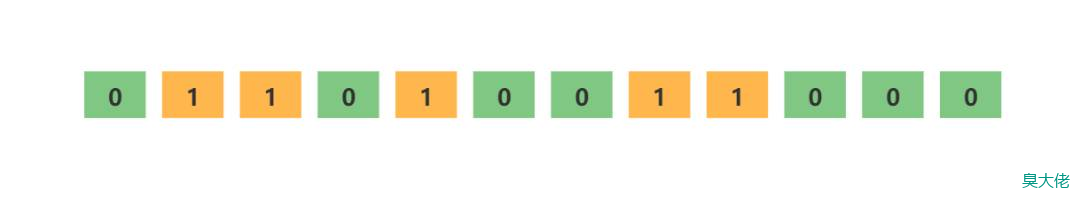##### 多个无偏hash函数：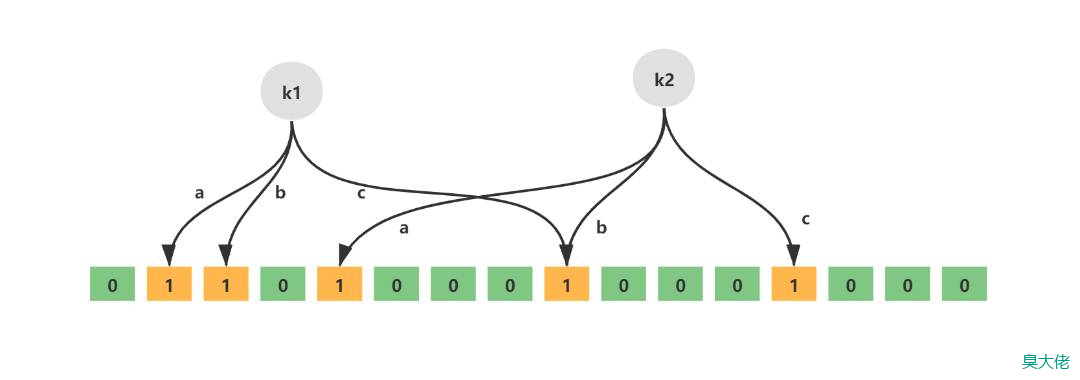#### 空间计算

• 错误率越低，位数组越长，控件占用较大
• 错误率越低，无偏hash函数越多，计算耗时较长

#### 增加元素

• 通过k个无偏hash函数计算得到k个hash值
• 依次取模数组长度，得到数组索引
• 将计算得到的数组索引下标位置数据修改为1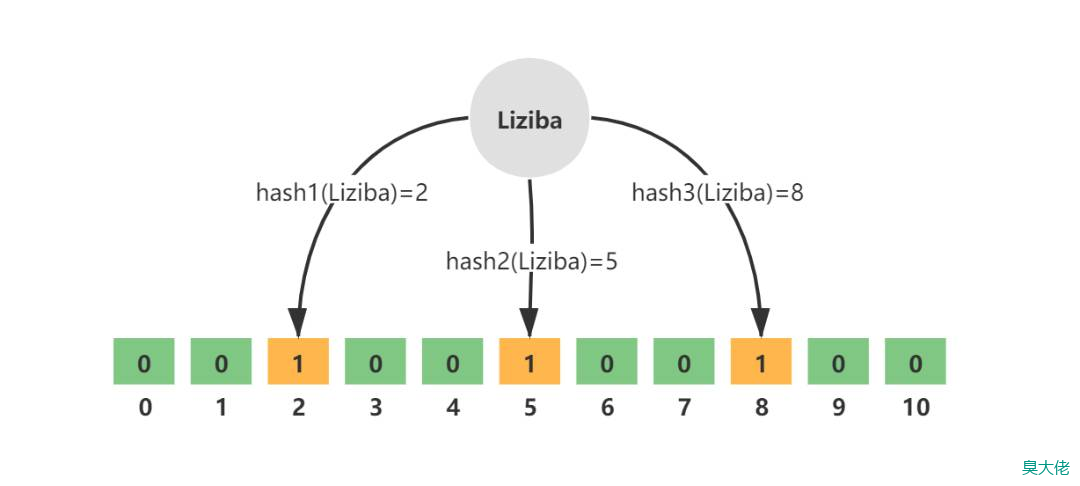#### 查询元素

• 通过k个无偏hash函数计算得到k个hash值
• 依次取模数组长度，得到数组索引
• 判断索引处的值是否全部为1，如果全部为1则存在（这种存在可能是误判），如果存在一个0则必定不存在
##### 关于误判

• 如果根据同一个哈希函数得到的哈希值不同，那么这两个哈希值的原始输入值肯定不同。
• 如果根据同一个哈希函数得到的两个哈希值相等，两个哈希值的原始输入值有可能相等，有可能不相等。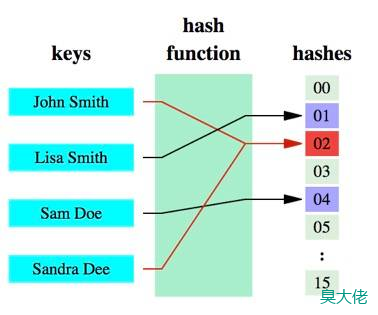## Redis 集成布隆过滤器

### 安装&编译

#### 下载

``````wget https://github.com/RedisBloom/RedisBloom/archive/refs/tags/v2.2.14.tar.gz
``````

#### 解压

``````tar -zxvf v2.2.14.tar.gz
``````

#### 编译

``````cd RedisBloom-2.2.14/
make
``````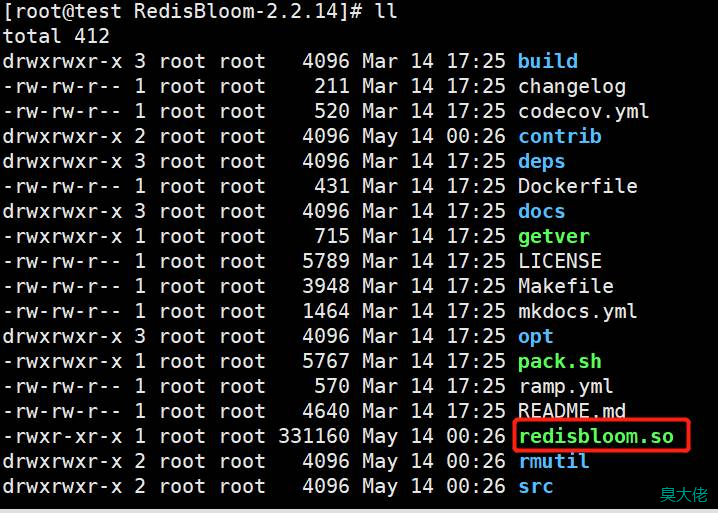#### 复制到redis目录

``````// 假设已经在 redis安装目录下

cp /root/RedisBloom-2.2.14/redisbloom.so redisbloom.so
``````

### Redis集成

``````loadmodule /www/server/redis/redisbloom.so
``````

#### 集成验证

``````redis-cli
``````

``````auth "你的redis密码"
``````

``````bf.exists user choudalao
``````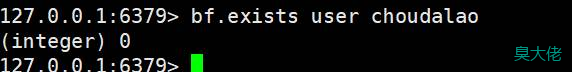## php-redis 布隆过滤器

BloomController

``````<?php
/**
* Description:
* User: Vijay <1937832819@qq.com>
* Site: https://www.choudalao.com/
* Date: 2022/5/14
* Time: 1:02
*/

namespace App\Http\Controllers\Api;

use App\Libs\BloomFilter;
use Predis\Client;
use App\Http\Controllers\Controller;

class BloomController extends Controller
{
protected \$redis = null;

public function __construct()
{
// 这是laravel的redis连接方式，根据自己框架修改就行
\$config = config('database.redis.default');
\$this->redis = new Client(\$config);
}

/**
* 向布隆过滤器压入字符串
* 这里使用了两个hash函数
*
* @return false|string
*/
public function put()
{
\$redis = \$this->redis;
\$HashFunctionArr = ['JSHash', 'PJWHash'];
\$RedisKey = 'test';
\$BloomLength = 8;//设置布隆位阵列的长度，hash后的数值对其取余
\$BloomFilter = new BloomFilter(\$redis, \$HashFunctionArr, \$RedisKey, \$BloomLength);
\$res = \$BloomFilter->put('joker');
return json_encode(\$res);
}

/**
* 判断是否存在
*
* @return false|string
*/
public function exists()
{
\$redis = \$this->redis;
\$HashFunctionArr = ['JSHash', 'PJWHash'];
\$RedisKey = 'test';
\$BloomLength = 8;//设置布隆位阵列的长度，hash后的数值对其取余
\$BloomFilter = new BloomFilter(\$redis, \$HashFunctionArr, \$RedisKey,\$BloomLength);
\$res = \$BloomFilter->isExists('joker');

return json_encode(\$res);
}

}
``````

BloomFilter

``````<?php
/**
* Description:
* User: Vijay <1937832819@qq.com>
* Site: https://www.choudalao.com/
* Date: 2022/5/14
* Time: 1:15
*/

namespace App\Libs;

use Predis\Client as Redis;

class BloomFilter
{
// hash 函数数组
public \$HashFunctionArr;
public \$Redis;
public \$RedisKey;
public \$BloomLength;

public function __construct(Redis \$redis, Array \$HashFunctionArr, string \$RedisKey, int \$BloomLength = 0)
{
\$this->HashFunctionArr = \$HashFunctionArr;
\$this->Redis = \$redis;
\$this->RedisKey = \$RedisKey;
\$this->BloomLength = \$BloomLength;
}

/**
* 添加 : 将哈希函数计算后的数字，在二进制对应位置置为1
* 返回 : hash处理后的数字
*
* @param string \$string
* @return mixed
*/
public function put(string \$string)
{
\$arr = [];
foreach (\$this->HashFunctionArr as \$function) {
\$arr[] = \$hash = \$this->BloomLength == 0 ?
BloomFilterHash::\$function(\$string) :
BloomFilterHash::\$function(\$string) % \$this->BloomLength;
\$this->Redis->setBit(\$this->RedisKey, \$hash, 1);
}

return \$arr;

}

/**
* 查询是否存在
*  1,存在的一定会存在
*  2,不存在有一定几率会误判
*
* @param string \$string
* @return bool
*/
public function isExists(string \$string)
{
\$len = strlen(\$string);
\$res = [];
foreach (\$this->HashFunctionArr as \$function) {
\$hash = \$this->BloomLength == 0 ?
BloomFilterHash::\$function(\$string, \$len) :
BloomFilterHash::\$function(\$string, \$len) % \$this->BloomLength;
\$res[] = \$this->Redis->getBit(\$this->RedisKey, \$hash);
}
foreach (\$res as \$bit) {
if (\$bit == 0) {
return false;
}
}
return true;
}
}
``````

BloomFilterHash

``````<?php
/**
* Description:
* User: Vijay <1937832819@qq.com>
* Site: https://www.choudalao.com/
* Date: 2022/5/14
* Time: 1:20
*/

namespace App\Libs;

class BloomFilterHash
{
/**
* 由Justin Sobel编写的按位散列函数
*/
public static function JSHash(\$string, \$len = null)
{
\$hash = 1315423911;
\$len || \$len = strlen(\$string);
for(\$i = 0; \$i < \$len; \$i++)
{
\$hash ^= ((\$hash << 5) + ord(\$string[\$i]) + (\$hash >> 2));
}
return (\$hash % 0xFFFFFFFF) & 0xFFFFFFFF;
}

/**
* 该哈希算法基于AT＆T贝尔实验室的Peter J. Weinberger的工作。
* Aho Sethi和Ulman编写的“编译器（原理，技术和工具）”一书建议使用采用此特定算法中的散列方法的散列函数。
*/
public static function PJWHash(\$string, \$len = null)
{
\$bitsInUnsignedInt = 4 * 8; //（unsigned int）（sizeof（unsigned int）* 8）;
\$threeQuarters = (\$bitsInUnsignedInt * 3) / 4;
\$oneEighth = \$bitsInUnsignedInt / 8;
\$highBits = 0xFFFFFFFF << (int)(\$bitsInUnsignedInt - \$oneEighth);
\$hash = 0;
\$test = 0;
\$len || \$len = strlen(\$string);
for(\$i = 0; \$i < \$len; \$i++)
{
\$hash = (\$hash << (int)(\$oneEighth)) + ord(\$string[\$i]);
}
\$test = \$hash & \$highBits;
if(\$test != 0)
{
\$hash = ((\$hash ^ (\$test >> (int)(\$threeQuarters))) & (~\$highBits));
}
return (\$hash % 0xFFFFFFFF) & 0xFFFFFFFF;
}

/**
* 类似于PJW Hash功能，但针对32位处理器进行了调整。它是基于UNIX的系统上的widley使用哈希函数。
*/
public static function ELFHash(\$string, \$len = null)
{
\$hash = 0;
\$len || \$len = strlen(\$string);
for(\$i = 0; \$i < \$len; \$i++)
{
\$hash = (\$hash << 4) + ord(\$string[\$i]);
\$x = \$hash & 0xF0000000;
if(\$x != 0)
{
\$hash ^= (\$x >> 24);
}
\$hash &= ~\$x;
}
return (\$hash % 0xFFFFFFFF) & 0xFFFFFFFF;
}

/**
* 这个哈希函数来自Brian Kernighan和Dennis Ritchie的书“The C Programming Language”。
* 它是一个简单的哈希函数，使用一组奇怪的可能种子，它们都构成了31 .... 31 ... 31等模式，它似乎与DJB哈希函数非常相似。
*/
public static function BKDRHash(\$string, \$len = null)
{
\$seed = 131;  # 31 131 1313 13131 131313 etc..
\$hash = 0;
\$len || \$len = strlen(\$string);
for(\$i = 0; \$i < \$len; \$i++)
{
\$hash = (int)((\$hash * \$seed) + ord(\$string[\$i]));
}
return (\$hash % 0xFFFFFFFF) & 0xFFFFFFFF;
}

/**
* 这是在开源SDBM项目中使用的首选算法。
* 哈希函数似乎对许多不同的数据集具有良好的总体分布。它似乎适用于数据集中元素的MSB存在高差异的情况。
*/
public static function SDBMHash(\$string, \$len = null)
{
\$hash = 0;
\$len || \$len = strlen(\$string);
for(\$i = 0; \$i < \$len; \$i++)
{
\$hash = (int)(ord(\$string[\$i]) + (\$hash << 6) + (\$hash << 16) - \$hash);
}
return (\$hash % 0xFFFFFFFF) & 0xFFFFFFFF;
}

/**
* 由Daniel J. Bernstein教授制作的算法，首先在usenet新闻组comp.lang.c上向世界展示。
* 它是有史以来发布的最有效的哈希函数之一。
*/
public static function DJBHash(\$string, \$len = null)
{
\$hash = 5381;
\$len || \$len = strlen(\$string);
for(\$i = 0; \$i < \$len; \$i++)
{
\$hash = (int)((\$hash << 5) + \$hash) + ord(\$string[\$i]);
}
return (\$hash % 0xFFFFFFFF) & 0xFFFFFFFF;
}

/**
* Donald E. Knuth在“计算机编程艺术第3卷”中提出的算法，主题是排序和搜索第6.4章。
*/
public static function DEKHash(\$string, \$len = null)
{
\$len || \$len = strlen(\$string);
\$hash = \$len;
for(\$i = 0; \$i < \$len; \$i++)
{
\$hash = ((\$hash << 5) ^ (\$hash >> 27)) ^ ord(\$string[\$i]);
}
return (\$hash % 0xFFFFFFFF) & 0xFFFFFFFF;
}

/**
* 参考 http://www.isthe.com/chongo/tech/comp/fnv/
*/
public static function FNVHash(\$string, \$len = null)
{
\$prime = 16777619; //32位的prime 2^24 + 2^8 + 0x93 = 16777619
\$hash = 2166136261; //32位的offset
\$len || \$len = strlen(\$string);
for(\$i = 0; \$i < \$len; \$i++)
{
\$hash = (int)(\$hash * \$prime) % 0xFFFFFFFF;
\$hash ^= ord(\$string[\$i]);
}
return (\$hash % 0xFFFFFFFF) & 0xFFFFFFFF;
}
}
``````

{{ comment.content }}@ {{ child.answered_user_name }}:{{ child.content }}• 微信• 如果你觉得本站很棒，可以通过扫码支付打赏哦！

•微信收款码
•支付宝收款码